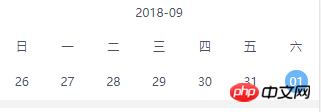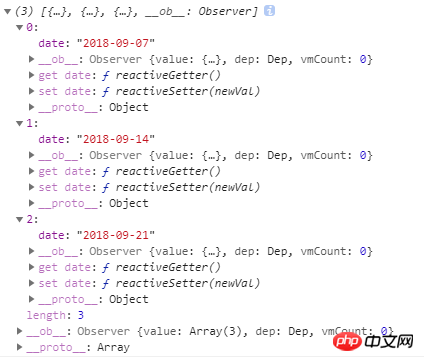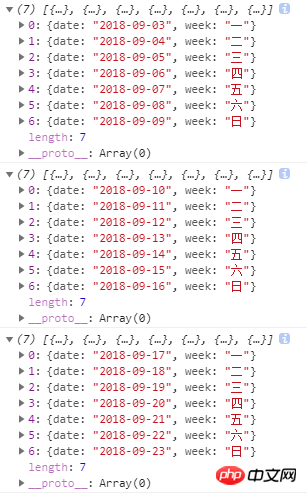# 如何基于moment实现日期可左右滑动的日历？1、先得到相邻三个周的数据，初始化的时候讲容器向左移动一个视口的距离，确保中间周在可视范围（在可是范围的所用为1）
2、触摸移动阶段，比如向左移动，相当于改变可是范围的索引，也就是2，即向左移动过两个视口的范围
3、移动结束，这时右边已经没有待显示的数据，需要重组数据，再向后加一周，使当前显示的周在中间，同时需要改变显示的索引为1

1、用moment处理日期数据

```let today = moment().format( YYYY-MM-DD ) // 当前日期： 2018-09-14
moment(today).subtract(7, d ).format( YYYY-MM-DD ) // 上一周的今天： 2018-09-07
moment(today).add(7, d ).format( YYYY-MM-DD ) // 下一周的今天： 2018-09-21 `````` getDays: function (day) {
let arr = []
/* 计算传进来的日期为星期几 */
let weekOfDate = Number(moment(day).format( E ))
// 提前定义好的： this.week = [ 一 , 二 , 三 , 四 , 五 , 六 , 日 ]
for (let i = 0; i this.week.length; i++) {
arr.push(
date: moment(day).subtract(weekOfDate - i - 1, d ).format( YYYY-MM-DD ),
week: this.week[i]
return arr
}`````` template v-for= (item, index) in dates
p >这里，静态显示已经完成为组件添加滑动功能改写上方的页面渲染代码 p > // actIndex: 当前活动视图的缩影，初始为1，sliderWidth：视口的宽度， distan： {x:0, y: 0}: 触摸移动的距离
getTransform: function () {
this.endx = (-this.actIndex * this.sliderWidth) + this.distan.x
let style = {}
style[ transform ] = translateX( + this.endx + px)
// 这一条必须写，因为触摸移动的时候需要过渡动画，但是在动画结束重组数据的时候需要瞬间回到该去的位置，不能要过渡动画
style[ transition ] = this.isAnimation ? transform .5s ease-out : none
return style
}最后触摸时间处理： touchStart: function (e) {
this.start.x = e.touches.pageX
touchmove: function (e) {
// 这里需要过渡动画
this.isAnimation = true
this.distan.x = e.touches.pageX - this.start.x
// 需要移动的容器
let dom = this.\$refs.sliders
// 向左
this.endx = this.endx + this.distan.x
dom.style[ transform ] = translateX( + this.endx + px)
touchend: function (e) {
this.isAnimation = true
this.distan.x = e.changedTouches.pageX - this.start.x
// 向右
if (this.distan.x 0) {
this.direction = right
this.actIndex = 0
} else if (this.distan.x 0) {
this.direction = left
this.actIndex = 2
this.distan.x = 0
},过渡结束后重置容器位置// 过渡结束
transitionEnd: function () {
this.isAnimation = false
if (this.actIndex === 2) {
this.dates.push({
date: moment(this.dates[this.actIndex].date).add(7, d ).format( YYYY-MM-DD )
this.dates.shift()
this.actIndex = 1
}else if (this.actIndex === 0) {
this.dates.unshift({
date: moment(this.dates[this.actIndex].date).subtract(7, d ).format( YYYY-MM-DD )
this.dates.pop()
this.actIndex = 1
}相关推荐：Atitit.基于时间戳的农历日历历法日期计算在js中如何实现图片左右滑动以上就是如何基于moment实现日期可左右滑动的日历？的详细内容，其它编程语言
郑重声明：本文版权归原作者所有，转载文章仅为传播更多信息之目的，如作者信息标记有误，请第一时间联系我们修改或删除，多谢。
```